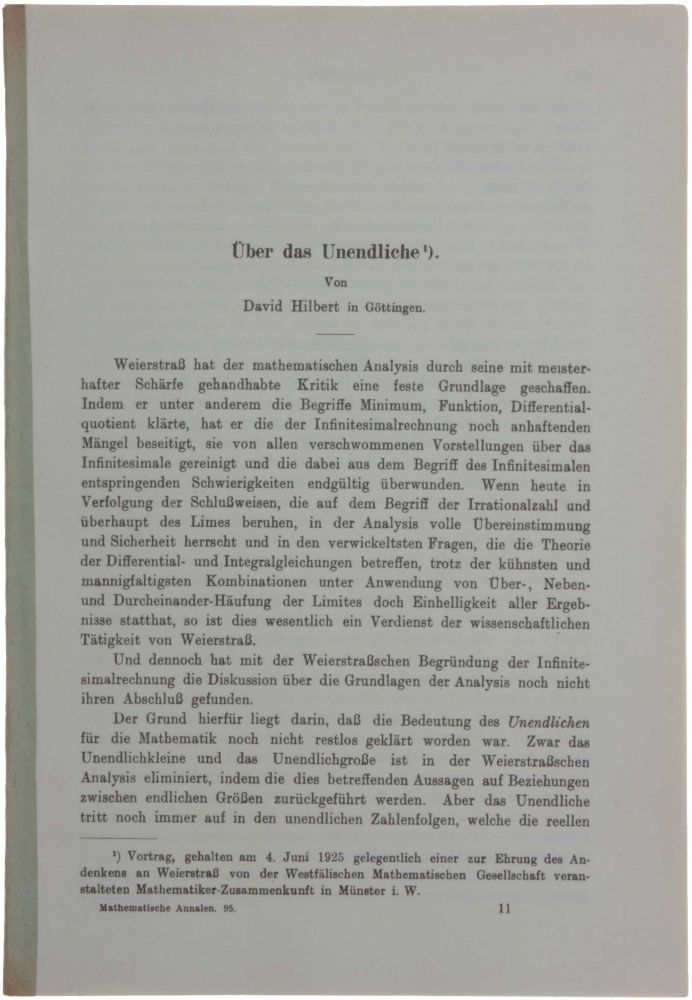## Über das Unendliche.

Berlin: Springer, 1925/1926.

Very rare separate printing (WorldCat locates just two copies) of the most detailed exposition of Hilbert’s proposal for the foundation of classical mathematics, which became known as Hilbert’s Program. This paper further contains his attempted proof of the continuum hypothesis which led to the later work of Kurt Gödel and Paul Cohen. (Van Heijenoort: From Frege to Gödel, pp.367-92:) “Hilbert devoted to the foundations of mathematics a series of papers ... Among all these contributions the 1925 paper [the offered item] stands out as the most comprehensive presentation of Hilbert’s ideas. It is the text of an address delivered in Münster on 4 June 1925 at a meeting organized by the Westphalian Mathematical Society to honor the memory of Weierstrass. In addition to the original version [ibid], two modified ones [were published in 1927 and 1930]. These, however, are abbreviated and besides other passages, omit entirely the section that deals with the continuum problem ... The paper consists of two quite distinct parts. The first is a clear and forceful presentation of Hilbert’s ideas at the time on the foundations of mathematics. It starts by recalling how Weiserstrass eliminated references to ‘infinity’ in analysis (Hilbert could have mentioned Cauchy and D’Alembert) and reviews the role played by infinite in physics, set theory, and, when we deal with general propositions, logic. Leaning on the example of arithmetic, it introduces the distinction between finitary and ideal propositions, and it undertakes a simultaneous formalization of logic and arithmetic ... The second part of the paper is a sketch of an attempted proof of the continuum hypothesis. Stated for the first time by Cantor at the very beginning of the development of set theory (1878), presented by Hilbert as Problem no. 1 in his famous list of unsolved mathematical problems (1900), the continuum problem for years resisted the efforts of mathematicians. The only partial result known at the time of Hilbert’s attempted proof was König's: the power of the continuum is not a limit of denumerably many smaller cardinal numbers ... Instead of the set of real numbers Hilbert considers the equivalent set of number-theoretic functions and undertakes to prove that there is a mapping of the set of ordinals of the second number class onto the set of these functions. By dealing with the definitions of functions rather than with the functions themselves the argument takes on a metamathematical aspect that is absent from Cantor’s work. The fact that the values of a number-theoretic function may be made to depend on the solvability of a certain mathematical problem establishes a connection between the second part of the paper and the first, and Hilbert invokes his previously expressed conviction that every well-posed mathematical problem is solvable. If the definition of a function involves the solvability of a mathematical problem, it will contain an unbounded existential quantifier, or, in Hilbert’s system, the ε-function. Convinced of the possibility of justifying the transfinite part of mathematics by metamathematical means, Hilbert states as Lemma I that such definitions of functions can be replaced by recursive definitions, in which, except for a string of prenex universal quantifiers, no quantifier occurs. ... Hilbert then proceeds to show that, since recursion equations defining functions contain ‘variable-types’ of increasing height, these equations parallel the definitions of the ordinals of the second number class. ... Among several unproved statements, it contains in particular, as Lemma II, the assertion that in the definitions of number-theoretic functions transfinite recursion can be replaced by ordinary recursion. Hilbert’s attempt aroused few comments. Paul Lévy recalls: ‘Zermelo told me in 1928 that even in Germany nobody understood what Hilbert meant’. ... The continuum hypothesis was proved by Gödel (1938, 1939, 1940) to be consistent with the customary axioms of set theory and by Cohen (1963-64) to be independent of these axioms. ... In his review of Gödel’s second paper of the consistency of the continuum (1939), Bernays wrote: ‘The whole Gödel reasoning may also be considered as a way of modifying the Hilbert project for a proof of the Cantor continuum hypothesis, as described in [Über das Unendliche, 1925], so as to make it practical and at the same time generalizable to higher powers’.”. See also Parkinson: Breakthroughs, p.199 and Bell: Men of Mathematics, p.63.

8vo: 226 x 157 mm. Offprint from: Mathematische Annalen, vol. 95, pp.  - 190. Bound with a light green backstrip as issued, a fine copy. Besides the two copies located by WorldCat in Germany and Holland, we could locate three other offprints; one in the Paul Hertz collection of the University of Pittsburgh, and two copies in a private collection.

Item #3181

Price: \$2,500.00